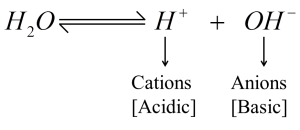Saturday, June 12, 2021
Home > Latest Announcement > Real Life Scenario of Logarithm: Acidic, Basic and Neutral Behavior of a Substance

# Real Life Scenario of Logarithm: Acidic, Basic and Neutral Behavior of a SubstanceDownloading Link is at Last

# Real life Application of Logarithm in pH values

In this post, you will learn about the real life application of Logarithm in pH values.

## Real Life scenario of Logarithms in Measuring pH Value

The Real Life scenario of Logarithms is to measure the acidic, basic or neutral of a substance that describes a chemical property in terms of pH value. To know this concept in detail of real life application of Logarithm in pH values, we start with pure water.

We know that Pure Water () is a very weak electrolyte. A small number of the molecules dissociate (split up) with each other.

The dissociation of water is into hydrogen ionsand hydroxide ions (OH). The hydrogen ionsis cations that are Acidic in nature and the hydroxide ions (OH) are anions that are Basic in nature.In pure water, there is an equal number of hydrogen ions and hydroxide ions. The solution is neither acidic or basic. It is in neutral form.

Since the dissociations take place to a very small extent, a constant of water (Kw) is created to show the equilibrium condition for the self-ionization of water as below.Thus, the product of concentrations ofandions in water at a particular temperature is known as the ionic product of water.

Now, in a laboratory, it is found that the value of= 1 × 10-14 M, at 25 degrees C. Since pure water is neutral in nature, the concentrations ofandions are equal.

Let== x.Thus,=M

Thus, in 1 liter, only 10–7 mole of water is in ionic form.

Now, when an acid (that donates hydrogen ions) is dissolved in water, the balance between hydrogen ions and hydroxide ions is shifted. There are more hydrogen ions than hydroxide ions in the solution. This kind of solution is acidic. Thus,>for the acidic solution.

When a base (that accepts hydrogen ions) is dissolved in water, the balance between hydrogen ions and hydroxide ions shifts the opposite way. There are more hydroxide ions than hydrogen ions in the solution. This kind of solution is alkaline. Thus,>for a basic solution.

 Acidic Neutral Basic (Alkaline)>=<> 1 × 10–7 M= 1 × 10–7 M< 1 × 10–7 M< 1 × 10–7 M= 1 × 10–7 M> 1 × 10–7 M

Now, it is concluded that every aqueous solution whether a solution is acidic, basic or neutral contains bothandions. Thus, the product of their concentration is always constant and equal to 1 × 10–14 M at 250C. If one of them increases then the other decreases so that the product remains same as 1 × 10–14 M.

For example:

• If= 10–2 M, then= 10–12 Thus, the product= (10–2 × 10–12) = 10–14 M
• If= 10–10 M, then= 10–4 Thus, the product= (10–10 × 10–4) = 10–14 M

We can say that the nature of the solution (Acidic, Basic or Neutral) can be found by hydrogen ions concentrationor hydroxyl ions concentration. But it is convenient to express acidity or basicity of solution by using by hydrogen ions concentration.

Now, we move to our concept as pH value, which is one of the real-life scenarios of logarithms. In 1909, the scientist Sorensen defines a pH (Potential or Power of Hydrogen) notation for hydrogen ions concentration. Since the hydrogen ion concentrations are expressed in terms of the negative power of 10, he defined this numerical value of negative power was termed as pH.

Thus, we have= 10–pH

Taking log both sides, we getlog= log (10–pH) = –pH log 10 = –pHpH = –log= logThus, pH of the solution is the negative logarithm of the concentration (in mol per litres) of hydrogen ions.Similarly, pOH of the solution is the negative logarithm of the concentration (in mol per liters) of hydroxyl ions. pOH = –log.

Now, we know that= 10–14

Taking log both sides, we get

log+ log= log(10–14) = –14 log10

(–log) + (–log) = 14

Thus, pH + pOH = 14.

Now, the previous table can be modified as below.

 Acidic Neutral Basic (Alkaline)>=<> 1 × 10–7 M= 1 × 10–7 M< 1 × 10–7 M< 1 × 10–7 M= 1 × 10–7 M> 1 × 10–7 M pH < 7 pH = 7 pH > 7 pOH > 7 pOH = 7 pOH < 7

Range of pH Scale:

According to pH Scale Principle, there are two results as below.
1. H
+ ion concentration and pH relate inversely

(a) Increasing pH means theions are decreasing.
(b) Decreasing pH meansions are increasing.

2. OH
ion concentration and pH relate directly

(a) Increasing pH meansions are increasing.
(b) Decreasing pH meansions are decreasing.

Now, it is clear that the pH values of a solution range from 0 to 14. Thus, the pH value from 0 to 14 is given below with the concentrations ofandand everyday examples.Litmus Paper to test the Acidity and Basicity of the solution:
Litmus paper is an indicator used to determine acidic, basic or neutral of a chemical. Since it is an indicator it can’t be used to determine the pH value of a solution, neither can the strength of the acidity or basicity be determined.
There are two types of litmus paper – red and blue.

Red litmus paper is used to test an alkaline/base. When a red litmus paper is immersed in a base or alkaline, the red litmus paper turns blue indicating the given solution as alkaline/base.
Blue litmus paper is used to test an acid. When a blue litmus paper immersed in an acid, the litmus paper turns red from blue indicating acid.

Following Experimental Labs to know the pH value of some chemicals.

error: Content is protected !!